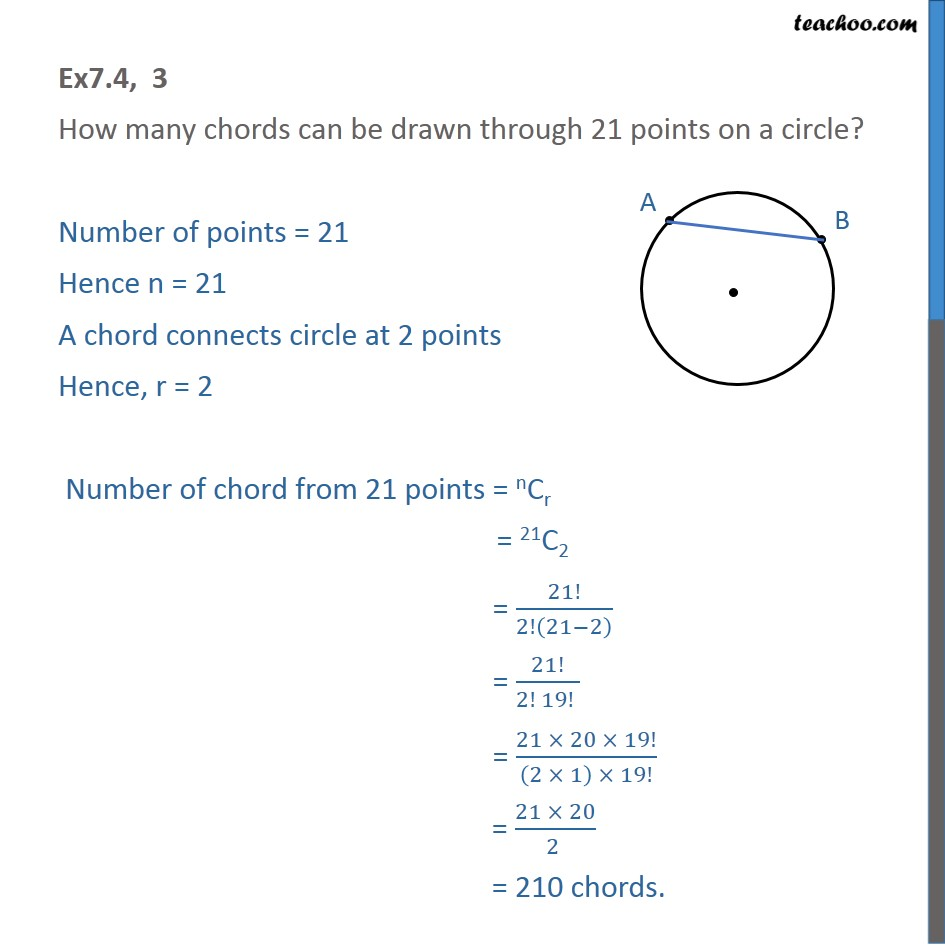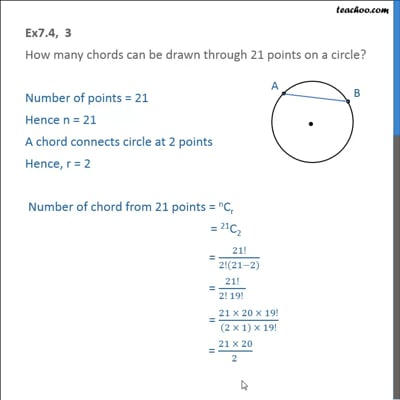Ex 7.4

Chapter 7 Class 11 Permutations and Combinations (Term 2)
Serial order wiseThis video is only available for Teachoo black users

### Transcript

Ex7.4, 3 How many chords can be drawn through 21 points on a circle? Number of points = 21 Hence n = 21 A chord connects circle at 2 points Hence, r = 2 Number of chord from 21 points = nCr = 21C2 = 21!/2!(21−2) = 21!/(2! 19! ) = (21 × 20 × 19!)/((2 × 1) × 19!) = (21 × 20)/(2 ) = 210 chords.